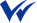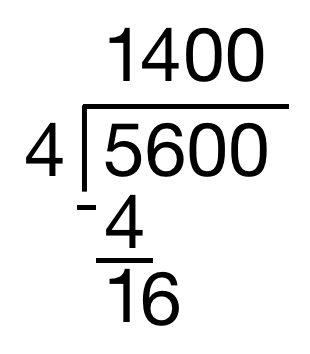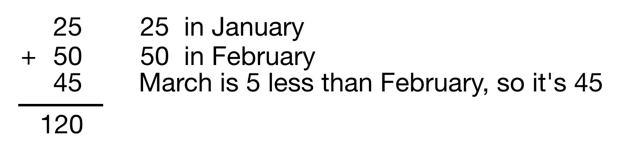# Ultimate Wonderlic/Wonscore Test Prep, May 2022

## Pass ANY Wonderlic Test With This Best Selling 3 Hours Easy Video-Based Crash Course

Bestseller 4.7 (106 ratings) 7,576 students

###### Created by :Michael Lerner, BSc, Psychometric Tutor, Wonderlic Test Expert

Within 3 hours of watching short videos and practicing online, you will be able to quickly answer ALL Wonderlic question types, avoid the notorious Wonderlic time traps, perfectly manage your time and Improve Your Score By 10 POINTS

#### This is the only prep that covers ALL Types of Wonderlic tests:

Wonscore | Wonderlic Personnel Test (WPT) | Wonderlic Scholastic Level Exam (SLE) Wonderlic Basic Skills Test (WBST) | Wonderlic Personality Test | Wonderlic Motivation Test

#### What you'll quickly learn

•Strategy and Time management - With 50 questions and only 12 minutes to answer them, an effective approach to answering questions is essential.
•Recognizing and Avoiding time traps - The Wonderlic test was made to catch test-takers out with disproportionately time-consuming questions, so it is vital that you know how to avoid these.
•Lighting fast tactics - There are 24 question types on the Wonderlic test. This course offers the optimal strategy for answering every one of these questions.
•How to approach the personality and motivation assessments - Each company or school is looking for different traits in candidates. Learn how to effectively showcase these traits to prospective employers / schools
##### Be fully prepared for the Test in Only 3 Hours
\$64.90 \$138 53% OFF•Lifetime access
•17 Instructional videos
•19 Written guides
•24 Types of questions explained with a unique tactic for each
•3 Additional full-length timed mode sample tests
•500 Practice questions with explanations
•Bonus personality and motivation assessment prep module
•Perfect for Wonderlic WonScore and Wonderlic SLE (Academic/ Nursing Schools)
•Perfect for Queensland Police Recruiting

## How is this course structured?

•Intro and strategy module - Video tutorial about the crucial time management strategy, guessing and skipping time trap questions and when you should use the scrap paper (spoiler alert - you barely need to use it.)
•Cognitive ability modules - There are 20 modules that cover each type of the Math, Logic, Verbal and General Knowledge questions you will encounter on the test.
•Practice questions - In each module there is a pinpoint 4-7 minutes tutorial video that explains the tactics and approaches needed to solve that specific type of question, and 15-25 practice questions.
•3 full length mock tests - At the end of the Cognitive Ability section, there are 3 full length timed practice tests used to mimic a real test environment and to make sure you know how to implement all you have learned in the course.
•Personality and Motivation Assessment preparation - 3 preparation modules for Wonderlic`s Personality and Motivation assessment to maximize your chances of passing the entire assessment.

## Averages – Written Guide

Topic Progress:

Questions with averages may consume time, and the challenge is to make these questions easy and turn them into quick points rather than making you waste precious time.

In this module, you will get acquainted with all types of averages questions you may see on the Wonderlic test.

You will learn an easier and faster approach to get the right answer for each type, and it’s not just a simple sum and divide solution.

In reality, the Wonderlic test writers create questions with averages that can be solved quickly without calculating the sum and then dividing it.

They expect sharp-eyed test takers to notice it.

You may need to use a simple sum and divide approach only on a few types of questions, and these questions are easy to calculate since these are with round numbers.

So, let’s take a look at the first type:

## Average Pivot

“Driving for Miles”

#### A taxi driver drove 30 miles on Monday, 50 miles on Tuesday, 60 miles on Wednesday, 70 miles on Thursday, and 90 miles on Friday. The average number of miles he drove per day was ___?___.

1. 60 miles
2. 55 miles
3. 70 miles
4. 65 miles
5. 74 miles

Now, you may instinctively rush in calculating the sum and then divide it by 5 days, but this a long way to go that may cause time loss and confusion.

When you take a look at the numbers, imagine that the numbers are set on an axis:

30__(40)__ 50__ 60__ 70__(80) __ 90

This series of numbers is symmetrical and finding the average of a symmetrical series is very easy.

Read on and I will explain what it means.

Let’s start by looking at the first and last numbers.

30 is at the same “distance” from 50 as 90 is from 70

30__(40)__ 50__ 60__ 70__(80) __ 90

Now let’s look at the inner numbers. 50 is at the same distance from 60 as 70 is.

30__(40)__ 50__ 60__ 70__(80) __ 90

This is an easy example to explain and demonstrate how the middle number in a 5-number symmetrical series is actually the average.

30__(40)__ 50__ 60__ 70__(80) __ 90

Another similar but trickier type is the hidden average pivot :

## Average Pivot

“Bicycle Units”

A bicycle factory manufactured 420 units in March, 440 units in April, 500 units in May and 520 units in June. The average number of units per month was ____?_____.
1. 465
2. 470
3. 450
4. 480
5. 485

These questions are with only a 4-number series and look more complicated since there is no visible average pivot.

Let’s see it again on an axis:

420_ 430_440_450 _460 _470 _480 _490 _500 _510 _520

Notice that the first number, 420, is at the same distance from 440, as the last number, 520, is at the same distance from 500.

420_ 430_440_450 _460 _470 _480 _490 _500 _510 _520

Looking at the first and last numbers, or the highest and lowest in a number series is a quick way to determine whether a series of numbers is symmetrical.

In the case of a 4-number series, it’s definitely a symmetrical series.

If it’s a 5-number series, it’s probably symmetrical, you just need to confirm it with the 3 inner numbers.

So, back to the example, there are 4 numbers in a symmetrical series, so 420 and 520 neutralize each other, which makes calculating the average very easy since you need to take into consideration only the two inner numbers – 440 and 500.

420_ 430_440_450 _460 _470 _480 _490 _500 _510 _520

From here on it’s very easy to determine that 470 is the average

440_450 _460 _470 _480 _490 _500

If we will stick to the “looking at the distance” approach, 470 is the number right in the middle with the same distance from 440 and 500.

If the number series in the question is not symmetrical, you will need to calculate it in your head or use the scrap paper like in this example:

## Unsymmetrical Number Series Averages

“Package Deliveries”

In a 4-day period, a delivery person delivered the following number of packages per day: 1150, 1200, 1900, and 1350. The average number of packages he delivered per day was __?__.
1. 1450
2. 1550
3. 1400
4. 1300
5. 1500

There is no shortcut for that question. You can see that the number series is not symmetrical by looking at the highest and lowest numbers.

1150_1200_1250_1300_1350_1400_1450_1500_1550_1600_1650_1700_1750_1800_1950_1900

The distances from the inner numbers are not the same

1150_1200_1250_1300_1350_1400_1450_1500_1550_1600_1650_1700_1750_1800_1950_1900

Therefore, you will have to calculate the old fashion way of sum and divide. Some test takers can do it in their head. Try the exercise questions in this module to see how you perform doing it without a scrap paper. If you feel more comfortable to do it on a scrap paper, then do it. The worst scenario is trying to calculate it in your head, get confused, waste time, and stress yourself by trying to save a few seconds.

So, if you choose to use a scrap paper, this is a reminder of how to do it quickly:

First, sum the numbers :

1200 + 1900 = 3100

1350 + 1150 = 2500

3100 + 2500 = 5 6 0 0

The sum is 5600.

Divide it by 4.Unlike the sum calculation before with a series of 4-digit numbers, try to do simple calculations like this in your head on the Wonderlic test.

Use the scrap paper only when it’s necessary.

In conclusion, the unsymmetrical number series average questions will take longer than 10 seconds but it can be an easy point.

If you are a bit stressed with calculations and you think it will make you waste time, consider guessing or mark the number of question on the scrap paper to come back to later if you will have the time to do so.

Remember that there are easier questions further on.

Here is another type of question that needs to be calculated:

## Number Series with Relations

“Eddie’s Grocery Expenses”

#### Eddie’s total grocery expenses in January was \$25. In February, it was \$25 more than that in January, and in March, it was \$5 less than his February cost. What was his average grocery expenses from January to March per month?

1. \$35
2. \$45
3. \$40
4. \$50
5. \$30

In these questions, there is no trick.

You need to avoid confusion and time loss.

These questions are built to be calculated the old fashioned way; therefore, the numbers in these questions are easy.

Again, some test takers can easily do it in their head, but we recommend always using the scrap paper on these questions to avoid confusion and mistakes since the relations between the numbers may be confusing.

Just do it step by step: write down the number for each month, sum it, and divide it by 3.

It may take a bit longer than 10 seconds, but if you quickly do it step by step, you got yourself an easy point.

So let’s write it on a scrap paper.120/3 = 40

That was smooth and easy.

It may look simple, but under the pressure of a real test, you may be confused, make mistakes, and lose time.

## Weekdays and Weekends Averages

#### Here is an example of a Weekdays and Weekends Averages question:

“Restaurant Waiters”

#### A restaurant employed 65 waiters per day from Monday to Friday, and 30 waiters per day on Saturday and Sunday. The average number of waiters employed per day was __?__

1. 45
2. 55
3. 50
4. 40
5. 35

The formula here is simple, and once you are familiar with it, it’s quick and easy.

Multiply the number per day on weekdays by 5 and then multiply the number per day on the weekends by 2, and sum it together.

Then divide the sum by 7 to get the right answer.

65 x 5 = 325
30 x 2 = 60

The sum is 385.

Use the scrap paper if you need it

Now, in this example, the number 385 is not divided by 7 intuitively to round numbers, like 350 or 420 for example.

In these cases, the average will end with 5, such as 55 in this example.

In order to quickly find the answer to these question, without using the scrap paper and wasting time, get yourself familiar with these common numbers multiplied by 7.

You will probably be more familiar with 140, 210, 280 etc. as these numbers divide by 7 with whole round numbers, but it’s a good idea to remember the multiplying in between.

Here is a table that shows certain products of 7.

7*10 = 70
7*15 = 105
7*20 = 140
7*25 = 175
7*30 = 210
7*35 = 245
7*40 = 280
7*45 = 315
7*50 = 350
7*55 = 385
7*60 = 420
7*65 = 455
7*70 = 490
7*75 = 525
7*80 = 560
7*85 = 595
7*90 = 630
7*95 = 665
7*100 = 700